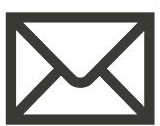Christoph Lenz Dipl.-Ing. Publications### Biography

Christoph Lenz was born in 1988 in Vienna, Austria. He studied Technical Mathematics at the Technische Universität Wien where he received his Bachelor's degree (2017) and the degree of Diplomingenieur (2019). After finishing his thesis which was written in collaboration with the IuE, he decided to join the research team of the institute, where he is currently working on his doctoral degree. His research interests include high performance algorithms and data structures and he will be working within the scope of the Christian Doppler Laboratory for High Performance TCAD.

## Automatic Feature Detection Methods for Implicit Surfaces

Automatic feature detection is a powerful tool to speed up the simulation of the fabrication of semiconductor devices, based on the level-set method. It allows one to focus valuable computational resources on parts of the simulation domain where high accuracy is of immense importance, while keeping the overall simulation runtime low. The general idea of all feature detection methods is to track the change in the direction of the surface normal when it moves along a surface. This can be achieved by the following two approaches. The first approach calculates all normal vectors of an implicit surface and then compares the angle between each normal vector and its adjacent normal vectors with a supplied threshold angle to decide if the surface point is a feature. In three dimensions, this approach requires a 27-point stencil around each surface point.

The other approach uses the mean curvature of an implicit surface. The mean curvature is an intrinsic property of orientable surfaces and originates from the field of differential geometry. The mean curvature of an implicit three-dimensional surface can be calculated by using the mean curvature formula for implicit functions, which requires a 19-point stencil around each surface point. If the implicit surface additionally fulfills the signed-distance property, the trace of the Hessian (shape operator) of the implicit surface can be used to approximate the mean curvature. This approach only requires a 7-point stencil around each surface point.

Fig. 1 shows the mean curvature values calculated with the mean curvature formula for implicit functions on a surface extracted during the simulation of the fabrication of stacked nanowires. To decide which of the calculated mean curvature values are to be considered as features, the absolute values of the mean curvatures are compared to a supplied threshold parameter.

The parallelization of the feature detection methods discussed above is straightforward. Fig. 2 shows the overall calculation time of these methods on a trench geometry where the parameters of all methods where chosen in such a way that they detect the same features.Fig. 1: Mean curvature values of a geometry extracted during the simulation of the fabrication process of stacked nanowires.Fig. 2: Parallel runtime of the discussed feature detection methods for a trench geometry.